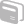# Activities

•##### Download

•• ##### Subject Area

• Math: Algebra I: Exponential Functions

• ##### Author9-12

45 Minutes

• ##### Device
•TI-Nspire™ CX/CX II
•TI-Nspire™ CX CAS/CX II CAS
• TI-Nspire™
• TI-Nspire™ CAS
• ##### Software

TI-Nspire™
TI-Nspire™ CAS

3.0

• ##### Report an Issue

Exponential Growth

#### Activity Overview

Model exponential growth and graph equations of the form y = a*bx.

#### Objectives

• Model exponential growth
• Graph equations of the form y = a*bx
• Solve problems using exponential equations
• Solve problems using compound interest
• Solve problems involving exponential decay

#### About the Lesson

Students will use Graphs, and Lists and Spreadsheets to graph, solve, and write two-variable linear inequalities. They will also solve systems of two-variable linear inequalities.

© Shell Education. TI-Nspire Strategies: Algebra (SEP50308), www.shelleducation.com